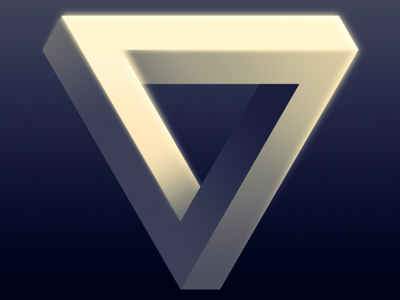Here is an impossible triangle - just for fun.

# Shapes - Perimeter and Area 2

This Math quiz is called 'Shapes - Perimeter and Area 2' and it has been written by teachers to help you if you are studying the subject at middle school. Playing educational quizzes is a fabulous way to learn if you are in the 6th, 7th or 8th grade - aged 11 to 14.

It costs only \$12.50 per month to play this quiz and over 3,500 others that help you with your school work. You can subscribe on the page at Join Us

Can you work out the perimeter or area of shapes such as a trapezium and a rhombus? The following Math quiz provides a more advanced look at area and perimeters of shapes.

Before trying this quiz, you might want to refresh your memory by playing the easier quiz called 'Level 3-4 Perimeter and Area 01'.

1.
In many formulas you will see the letter h which stands for 'height'. What would be a more correct term for 'height'?
Perpendicular height
Perpetrator height
Perpetual height
Perplexing height
The perpendicular height of an object should not be confused with the length of its shortest side
2.
If a parallelogram has a perpendicular height of 7 cm and a base of 11 cm, what is its area?
49 cm2
121 cm2
77 cm2
It is impossible to say
The formula for calculating the area of a parallelogram is A = bh (Area = base x height)
3.
What is the area of a triangle that has a base of 37 cm and a perpendicular height of 28 cm?
518 cm2
1,063 cm2
1,360 cm2
1,630 cm2
Area of a triangle = 12(b x h)
4.
If a parallelogram has a perpendicular height of 7 cm and a base of 11 cm, what is its perimeter?
44 cm
28 cm
36 cm
It is impossible to say
Did you get it wrong by confusing the perpendicular height with the length of its shortest side? The perpendicular height is NOT the same as the length of the shortest side!
5.
Archie says that the area of a rhombus of side length 12 cm is the same as that of a square of side 12 cm. Is he right?
Always
Never
Sometimes - it depends on the size of the internal angles
Only if the perimeter is equal to 48cm
If the rhombus has a right angle, it is also a square
6.
A garden is in the shape of a trapezium and it has an area of 1,500 meters2. One of the parallel sides is 40 meters long and the other parallel side is 60 meters long. What is the distance between the parallel sides?
20 meters
30 meters
40 meters
50 meters
When you are dealing with shapes it is often useful to draw the shape so that you can understand the question better
7.
If the area of a triangle is 420 cm2 and its perpendicular height is 30 cm, what is the length of its base?
24 cm
26 cm
28 cm
32 cm
8.
Is a square also a rectangle?
Yes
No
It depends on the length of its sides
It depends on its surface area
A square is a special rectangle - its length and width are the same
9.
The two parallel sides of a trapezium have lengths 10 cm and 20 cm, and the distance between them is 15 cm. What is the area of this trapezium?
150 cm2
200 cm2
225 cm2
250 cm2
Area of a trapezium = 12 (a + b)h
10.
If a rhombus has a base of 1.2 meters and a perpendicular height of 0.8 meters, what is its area?
0.64 m2
0.80 m2
0.96 m2
1.44 m2
Area of a rhombus = perpendicular height x side length
Author:  Frank Evans